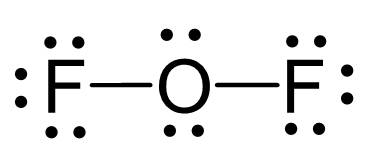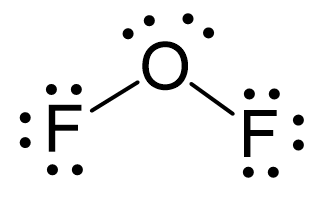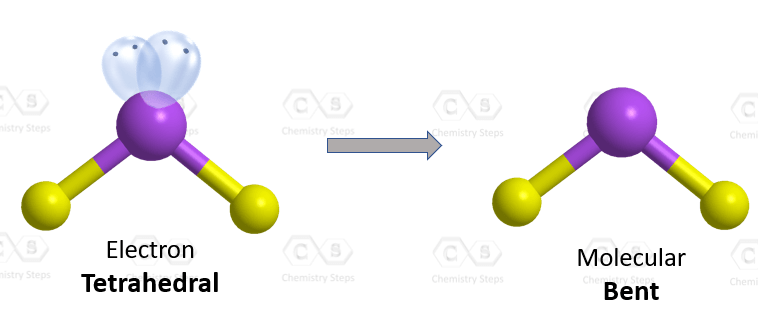## Examples

Oxygen is the central atom, so we can draw a preliminary skeletal structure:There are 6 + 2×7 = 20 electrons, and 4 of them are used to make 2 bonds. The two fluorines take 6 lone pairs, and the remaining four electrons go to the sulfur:The central atom has a steric number of 4 – two atoms and two lone pairs. The electron geometry, therefore, is tetrahedral, and the molecular geometry is bent.Steric number 4 corresponds to sp3-hybridization where the idealized bond angles are 109.5o. Because the groups on the central atom are not identical, the bond angles differ from the idealized values.

Check Also